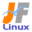Linux Kernel 2.6 Documentation: /usr/src/linux/Documentation/usb/hotplug.txt

usb/hotplug.txt

USB \$B%[%C%H%W%i%0\$N@bL@(B [\$B%W%l%\$%s%F%-%9%HHG(B]
• \$B86Cx:n
• \$BK]Lu
• \$B%P!<%8%g%s(B: 2.6.12
• \$BK]LuF|;~(B: 2005/08/17
```

==================================
\$B\$3\$l\$O!"(B
linux-2.6.13-rc3/Documentation/usb/hotplug.txt \$B\$NOBLu(B
\$B\$G\$9!#(B
\$BK]LuCDBN!'(B JF \$B%W%m%8%'%/%H(B < http://www.linux.or.jp/JF/ >
\$BK]LuF|(B \$B!'(B 2005/8/2
\$BK]LuuBV\$G%G%P%\$%9\$r%P%9\$K@\B3\$7\$^\$9!#\$[\$H\$s\$I\$N>l9g!"%f!<%6\$O\$9\$0\$K%G%P%\$%9(B
\$B\$,;H\$(\$k\$h\$&\$K\$J\$C\$FM_\$7\$\$\$H;W\$C\$F\$\$\$^\$9!#\$3\$l\$O!"%7%9%F%`\$,l9g\$b\$"\$j\$^\$9!#(B

This involves a mix of kernel mode and user mode actions.  Making devices
be immediately usable means that any user mode actions can't wait for an
administrator to do them:  the kernel must trigger them, either passively
(triggering some monitoring daemon to invoke a helper program) or
actively (calling such a user mode helper program directly).

\$B\$3\$l\$K\$O!"%+!<%M%k%b!<%I\$H%f!<%6%b!<%I\$r:.9g\$7\$?F0:n\$,I,MW\$G\$9!#(B
\$B%G%P%\$%9\$r\$9\$0\$K;H\$(\$k\$h\$&\$K\$9\$k\$H\$\$\$&\$3\$H\$O!"\$I\$N\$h\$&\$J%f!<%6%b!<%IF0:n\$b!"(B
\$B\$=\$l\$i\$rF0\$+\$9\$?\$a\$K4IM}@\8F\$S=P\$9(B)
\$B\$I\$A\$i\$+\$K\$h\$C\$F!"\$=\$l\$i\$r5/F0\$7\$J\$1\$l\$P\$J\$j\$^\$;\$s!#(B

Those triggered actions must support a system's administrative policies;
such programs are called "policy agents" here.  Typically they involve
shell scripts that dispatch to more familiar administration tools.

\$B\$3\$N\$h\$&\$K5/F0\$5\$l\$?F0:n\$O!"%7%9%F%`\$N4IM}%]%j%7!<\$r%5%]!<%H\$7\$J\$1\$l\$P\$J\$j\$^\$;\$s(B;
\$B\$3\$3\$G\$O!"\$=\$N\$h\$&\$J%W%m%0%i%`(B(\$B\$?\$\$\$F\$\$\$N>l9g!"\$h\$/CN\$i\$l\$F\$\$\$k4IM}%D!<%k\$K%G%#%9%Q%C%A(B
\$B\$9\$k%7%'%k%9%/%j%W%H\$r4^\$_\$^\$9(B)\$B\$r!"(B"\$B%]%j%7!<%(!<%8%'%s%H(B"\$B\$H8F\$S\$^\$9!#(B

that is currently available only when the drivers are dynamically linked,

\$B\$3\$l\$iF0:n\$N\$\$\$/\$D\$+\$O!"%I%i%\$%P\$K4X\$9\$k>pJs(B(\$B%a%?%G!<%?(B)(\$B8=:_%I%i%\$%P\$,D>@\%j%s%/(B
\$B\$7\$F\$\$\$k\$H\$-\$@\$1MxMQ\$G\$-\$^\$9(B)\$B\$K0MB8\$9\$k\$b\$N\$b\$"\$k\$N\$G!"9bEY\$K%b%8%e!<%k2=\$5\$l\$?(B
\$B%7%9%F%`\$r9=@.\$9\$k\$H!":G9b\$N%[%C%H%W%i%04D6-\$rF@\$i\$l\$^\$9!#(B

KERNEL HOTPLUG HELPER (/sbin/hotplug)

\$B%+!<%M%k%[%C%H%W%i%0%X%k%Q!<(B (/sbin/hotplug)

When you compile with CONFIG_HOTPLUG, you get a new kernel parameter:
/proc/sys/kernel/hotplug, which normally holds the pathname "/sbin/hotplug".
That parameter names a program which the kernel may invoke at various times.

CONFIG_HOTPLUG \$B\$rAH\$_9~\$`\$H!"?7\$7\$\$%+!<%M%k%Q%i%a!<%?\$,;H\$(\$^\$9(B
/proc/sys/kernel/hotplug  (\$B\$3\$NFbMF\$ODL>o!"%Q%9L>(B "/sbin/hotplug" \$B\$K\$J\$j\$^\$9(B)
\$B\$=\$N%Q%i%a!<%?\$K\$O!"%+!<%M%k\$,IQHK\$K5/F0\$9\$k%W%m%0%i%`\$r;XDj\$7\$^\$9!#(B

The /sbin/hotplug program can be invoked by any subsystem as part of its
reaction to a configuration change, from a thread in that subsystem.
Only one parameter is required: the name of a subsystem being notified of
some kernel event.  That name is used as the first key for further event
dispatch; any other argument and environment parameters are specified by
the subsystem making that invocation.

/sbin/hotplug \$B%W%m%0%i%`\$O@_Dj\$rJQ99\$9\$k\$?\$a!"\$=\$N%5%V%7%9%F%`\$K\$"\$k%9(B
\$B%l%C%I\$+\$i!"\$=\$NH?1~\$N0lItJ,\$N\$h\$&\$J\$I\$s\$J%5%V%7%9%F%`\$K\$h\$C\$F\$b5/F0\$G(B
\$B\$-\$^\$9!#%Q%i%a!<%?\$O\$R\$H\$D\$@\$1I,MW\$G\$9(B:
\$B\$"\$k%+!<%M%k%\$%Y%s%H\$K\$D\$\$\$FDLCN\$r\$K\$J\$j\$^\$9!#(B
\$B\$=\$NL>A0\$O!"\$5\$i\$J\$k%\$%Y%s%H%G%#%9%Q%C%A\$G!":G=i\$N%-!<\$H\$7\$F;HMQ\$5\$l\$^\$9(B;
\$BB>\$N0z?t!"\$*\$h\$S!"4D6-JQ?t\$O!"%5%V%7%9%F%`5/F0;~\$K@_Dj\$5\$l\$^\$9!#(B

Hotplug software and other resources is available at:

\$B%[%C%H%W%i%0%=%U%H%&%'%"\$H!"B>\$N;q8;\$O!"l=j\$K\$"\$j\$^\$9(B:

http://linux-hotplug.sourceforge.net

Mailing list information is also available at that site.

\$B%a!<%j%s%0%j%9%H>pJs\$b\$=\$N%5%\$%H\$K\$"\$j\$^\$9!#(B

--------------------------------------------------------------------------

USB POLICY AGENT

USB \$B%]%j%7!<%(!<%8%'%s%H(B

The USB subsystem currently invokes /sbin/hotplug when USB devices
are added or removed from system.  The invocation is done by the kernel
hub daemon thread [khubd], or else as part of root hub initialization
(done by init, modprobe, kapmd, etc).  Its single command line parameter
is the string "usb", and it passes these environment variables:

\$B8=:_!"(BUSB \$B%5%V%7%9%F%`\$O!"(BUSB \$B%G%P%\$%9\$,IU\$130\$7\$5\$l\$?\$H\$-\$K!"(B/sbin/hotplug \$B\$r5/F0\$7\$^\$9!#(B
\$B5/F0\$O!"%+!<%M%k%O%V%G!<%b%s%9%l%C%I(B [khubd] \$B\$d!"%k!<%H%O%V=i4|2=\$N0lIt(B
(init, modprobe, kapmd, \$B\$J\$I(B) \$B\$K\$h\$jl9g!"(B DEVICE \$B\$H(B DEVFS \$B\$bEO\$7\$^\$9!#(B
DEVICE \$B\$O!"%G%P%\$%9\$N%Q%9L>\$G!"%I%i%\$%PA*Br\$r:\$Fq\$K\$9\$k!"J#?t(B \$B\$*\$h\$S(B/\$B\$^\$?\$O(B
\$BBeM}%\$%s%?%U%'!<%9\$r;}\$D%G%P%\$%9\$KM-8z\$G\$9!#;EMM\$G!"(BUSB \$B%[%C%H%W%i%0(B \$B\$O!"(B
"usbdevfs" \$B\$H\$O!"L54X78\$G\$9(B: USB \$B%G%P%\$%9\$N\$[\$H\$s\$I\$N4pK\E*@_Dj\$O!"(B
\$B\$=\$N%U%!%\$%k%7%9%F%`\$r;H\$&I,MW\$,\$"\$j\$^\$;\$s!#\$5\$i\$K!"%7%9%F%`@_Dj\$NJQ99\$r8!=P\$9\$k(B
\$B%f!<%6%b!<%I%G!<%b%s\$b\$l\$N%I%i%\$%P\$N!"(BMODULE_DEVICE_TABLE
\$B\$+\$i\$N%(%s%H%j\$r4^\$`!"(B"modules.usbmap" \$B%U%!%\$%k\$r:n@.\$7\$^\$9!#(B
\$B\$3\$N\$h\$&\$J%U%!%\$%k\$O!"%V!<%H;~\$d!"\$=\$l0J8e\$K!"@5\$7\$\$%I%i%\$%P%b%8%e!<%k\$,%m!<%I\$5\$l\$?\$N\$r(B
\$B3NG'\$9\$k\$?\$a\$K!"G\$0U\$N%f!<%6%b!<%I%]%j%7!<%(!<%8%'%s%H\$G;H\$(\$^\$9!#(B

See <linux/usb.h> for full information about such table entries; or look
at existing drivers.  Each table entry describes one or more criteria to
be used when matching a driver to a device or class of devices.  The
specific criteria are identified by bits set in "match_flags", paired
with field values.  You can construct the criteria directly, or with

\$B\$3\$N\$h\$&\$J%F!<%V%k%(%s%H%j\$K\$D\$\$\$F\$NA4>pJs\$O!"(B<linux/usb.h> \$B\$r8+\$F\$/\$@\$5\$\$(B;
\$B\$^\$?\$O!"B8:_\$9\$k%I%i%\$%P\$r8+\$F\$/\$@\$5\$\$!#\$=\$l\$>\$l\$N%F!<%V%k%(%s%H%j\$O!"(B
\$B%I%i%\$%P\$,%G%P%\$%9\$"\$k\$\$\$O%G%P%\$%9\$N%/%i%9\$K9g\$C\$F\$\$\$k\$J\$i;H\$o\$l\$k\$O\$:\$N!"(B
\$B\$R\$H\$D\$"\$k\$\$\$O\$=\$l0J>e\$N4p=`\$r5-=R\$7\$F\$\$\$^\$9!#(B
\$BFCDj\$N4p=`\$O!"(B"match_flags" \$BFb\$N%S%C%H%;%C%H\$H!"%U%#!<%k%ICM\$NBP\$G!"(B
\$B<1JL\$5\$l\$^\$9!#4p=`\$OD>@\!"\$^\$?\$O!"\$=\$N\$h\$&\$J%^%/%m\$G:n\$k\$3\$H\$,\$G\$-\$^\$9!#\$^\$?!"(B
\$B>\:Y>pJs\$rJ]B8\$9\$k\$?\$a\$K!"(Bdriver_info \$B\$r;H\$C\$F\$/\$@\$5\$\$!#(B

USB_DEVICE (vendorId, productId)
... matching devices with specified vendor and product ids
USB_DEVICE_VER (vendorId, productId, lo, hi)
... like USB_DEVICE with lo <= productversion <= hi
USB_INTERFACE_INFO (class, subclass, protocol)
... matching specified interface class info
USB_DEVICE_INFO (class, subclass, protocol)
... matching specified device class info

USB_DEVICE (vendorId, productId)
... \$B;XDj\$7\$?%Y%s%@\$H%W%m%@%/%H(BID\$B\$G!"%G%P%\$%9\$H>H9g\$9\$k(B
USB_DEVICE_VER (vendorId, productId, lo, hi)
... USB_DEVICE \$B\$HF1MM\$G!"(B lo <= productversion <= hi \$B\$,\$H\$b\$J\$&(B
USB_INTERFACE_INFO (class, subclass, protocol)
... \$B;XDj\$7\$?%\$%s%?%U%'!<%9%/%i%9>pJs\$G>H9g\$9\$k(B
USB_DEVICE_INFO (class, subclass, protocol)
... \$B;XDj\$7\$?%G%P%\$%9%/%i%9>pJs\$G>H9g\$9\$k!#(B

A short example, for a driver that supports several specific USB devices
and their quirks, might have a MODULE_DEVICE_TABLE like this:

\$B?te\$K=E\$M\$i\$l\$?%\$%s%?%U%'!<%9\$r;HMQ\$7\$F@\B3\$9\$k\$b\$N\$b\$"\$j!"(B
\$B\$=\$l\$i\$O\$3\$N\$h\$&\$J!"(B"struct usb_driver" \$B\$rITMW\$H\$7\$^\$9!#(B

Drivers that connect directly to the USB subsystem should be declared
something like this:

USB \$B%5%V%7%9%F%`\$KD>@\@\B3\$9\$k%I%i%\$%P\$O!"\$3\$N\$h\$&\$KDj5A\$7\$J\$1\$l\$P\$J\$j\$^\$;\$s(B:

static struct usb_driver mydriver = {
.name		= "mydriver",
.id_table	= mydriver_id_table,
.probe		= my_probe,
.disconnect	= my_disconnect,

/*
if using the usb chardev framework:
.minor		= MY_USB_MINOR_START,
.fops		= my_file_ops,
if exposing any operations through usbdevfs:
.ioctl		= my_ioctl,
*/

/*
usb chardev \$B%U%l!<%`%o!<%/\$r\$D\$+\$&\$J\$i(B:
.minor		= MY_USB_MINOR_START,
.fops		= my_file_ops,
usbdevfs \$B7PM3\$G\$9\$Y\$F\$NF0:n\$r8x3+\$9\$k\$J\$i(B:
.ioctl		= my_ioctl,
*/

}

When the USB subsystem knows about a driver's device ID table, it's used when
choosing drivers to probe().  The thread doing new device processing checks
drivers' device ID entries from the MODULE_DEVICE_TABLE against interface and
device descriptors for the device.  It will only call probe() if there is a
match, and the third argument to probe() will be the entry that matched.

USB \$B%5%V%7%9%F%`\$,%I%i%\$%P\$N%G%P%\$%9(B ID \$B%F!<%V%k\$rM}2r\$9\$k\$H!"\$=\$l\$O!"(Bprobe() \$B\$XEO\$9%I%i%\$%P\$r(B
\$BA*Br\$9\$k\$N\$K;H\$o\$l\$^\$9!#?7\$7\$\$%G%P%\$%9=hM}\$r9T\$&%9%l%C%I\$O!"%G%P%\$%9\$N\$?\$a\$N%\$%s%?%U%'!<%9\$H!"(B
\$B%G%P%\$%9%G%#%9%/%j%W%?\$KBP\$7\$F!"(BMODULE_DEVICE_TABLE \$B\$+\$i\$N%I%i%\$%P\$N%G%P%\$%9(B ID \$B%(%s%H%j\$r(B
\$B>H9g\$7\$^\$9!#0lCW\$7\$?>l9g!"\$=\$l\$O!"(Bprobe() \$B\$r8F\$S=P\$9\$@\$1\$G\$9!#\$^\$?!"(Bprobe() \$B\$KEO\$9(B3\$BHVL\\$N0z?t\$O!"(B
\$B0lCW\$7\$?%(%s%H%j\$K\$J\$j\$^\$9!#(B

If you don't provide an id_table for your driver, then your driver may get
probed for each new device; the third parameter to probe() will be null.

\$B%I%i%\$%P\$N\$?\$a\$N(B id_table \$B\$,\$J\$\$\$J\$i!"%I%i%\$%P\$O\$=\$l\$>\$l\$N?7\$7\$\$%G%P%\$%9\$N\$?\$a\$KD4::\$5\$l\$?J*\$rF@\$^\$9(B;
probe() \$B\$KEO\$9(B3\$BHVL\\$N%Q%i%a!<%?\$OL58z\$K\$J\$j\$^\$9!#(B
```